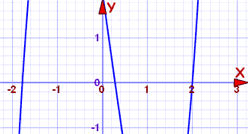Definition of

# Factor TheoremA polynomial's factors are related to its roots (where it equals zero) this way:

When f(c)=0 then x−c is a factor of f(x)

Likewise
When x−c is a factor of f(x) then f(c)=0